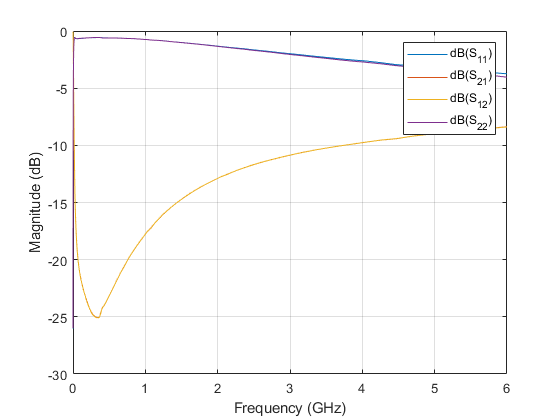Combine S-parameters to form cascade network

Description

example

s_params= cascadesparams(s1_params,s2_params,...,sk_params) cascades the scattering parameters (S-parameters) of K input networks described by the S-parameters. Each input network must be a 2N-port network described by a 2N-by-2N-by-M array of S-parameters for M frequency points. All networks must have the same reference impedance.

Note

The cascadesparams function uses ABCD-parameters. Alternatively, one can use S-parameters and ABCD-parameters (or T-parameters) to cascade S-parameters together by hand (assuming identical frequencies)

s_params= cascadesparams(___,Kconn) creates the cascaded networks based on the number of cascaded connections between the networks specified by Kconn. Use this option with the input arguments in the previous syntax.

hs= cascadesparams(hs1,hs2,...,hsk) cascades K S-parameter objects to create a cascade network. The function checks that the impedance and frequencies of each object is equal and that the parameters of each object contain 2N-by-2N-by-M array of S-parameters for M frequency points.

Examples

collapse all

Assemble a 2-port cascaded network from two sets of 2-port S-parameters operating at 2 GHz and at 2.1 GHz.

Create two sets of 2-port S-parameters.

freq = [2e9 2.1e9];
analyze(ckt1,freq);
analyze(ckt2,freq);
sparams_2p_1 = ckt1.AnalyzedResult.S_Parameters;
sparams_2p_2 = ckt2.AnalyzedResult.S_Parameters;

-0.4332 + 0.5779i   0.0081 - 0.0120i
2.6434 + 1.2880i   0.5204 - 0.5918i

-0.1271 + 0.3464i  -0.0004 - 0.0211i
3.8700 - 0.6547i   0.4458 - 0.6250i

Assemble a 3-port cascaded network from a set of 3-port S-parameters and a set of 2-port S-parameters.Create one set of 3-port S-parameters and one set of 2-port S-parameters.

freq = [2e9 2.1e9];
a1 = analyze(ckt1,freq);
a2 = analyze(ckt2,freq);
sparams_3p = sparameters(a1);
sparams_2p = sparameters(a2);

Cascade the two sets by connecting one port between them.

Kconn = 1;
sparameters: S-parameters object

NumPorts: 3
Frequencies: [2x1 double]
Parameters: [3x3x2 double]
Impedance: 50.0000 + 0.0000i

rfparam(obj,i,j) returns S-parameter Sij

Assemble a 3-port cascaded network from a set of 3-port S-parameters and a set of 2-port S-parameters, connecting the second port of the 3-port network to the first port of the 2-port.freq = [2e9 2.1e9];
a1 = analyze(ckt1,freq);
a2 = analyze(ckt2,freq);
sparams_3p = sparameters(a1);
sparams_2p = sparameters(a2);

Reorder the second and third ports of the 3-port network

sparams_3p_2 = snp2smp(sparams_3p,[1 3 2]);

Cascade the two sets by connecting one port between them

Kconn = 1;
sparams_2p,Kconn)
sparameters: S-parameters object

NumPorts: 3
Frequencies: [2x1 double]
Parameters: [3x3x2 double]
Impedance: 50.0000 + 0.0000i

rfparam(obj,i,j) returns S-parameter Sij

Assemble a 3-port cascaded network from a set of 3-port S-parameters and two sets of 2-port S-parameters.freq = [2e9 2.1e9];
a1 = analyze(ckt1,freq);
a2 = analyze(ckt2,freq);
a3 = analyze(ckt3,freq);
sparams_3p = sparameters(a1);
sparams_2p_1 = sparameters(a2);
sparams_2p_2 = sparameters(a3);

Connect one port between each set of adjacent networks.

Kconn = [1 1];
sparams_2p_1,sparams_2p_2,Kconn)
sparameters: S-parameters object

NumPorts: 3
Frequencies: [2x1 double]
Parameters: [3x3x2 double]
Impedance: 50.0000 + 0.0000i

rfparam(obj,i,j) returns S-parameter Sij

Assemble a 3-port cascaded network from a set of 3-port S-parameters and two sets of 2-port S-parameters, connecting the 3-port network to both 2-port networks.

freq = [2e9 2.1e9];
analyze(ckt1,freq);
analyze(ckt2,freq);
analyze(ckt3,freq);
sparams_3p = ckt1.AnalyzedResult.S_Parameters;
sparams_2p_1 = ckt2.AnalyzedResult.S_Parameters;
sparams_2p_2 = ckt3.AnalyzedResult.S_Parameters;

Cascade sparams_3p and sparams_2p_1 by connecting one port between them.

Kconn = 1;
sparams_2p_1, Kconn);

Reorder the second and third ports of the 3-port network.

50, [1 3 2]);

Cascade sparams_3p and sparams_2p_2 by connecting one port between them.

sparams_2p_2, Kconn)

0.1724 - 0.9106i   0.0240 + 0.0134i   0.0104 + 0.0971i
-3.7923 + 6.1234i  -0.7168 - 0.6498i  -0.5855 + 1.6475i
0.1214 + 0.0866i   0.0069 + 0.0090i   0.6289 - 0.6145i

-0.3014 - 0.6620i   0.0072 - 0.0255i  -0.0162 + 0.1620i
6.3709 + 2.2809i  -0.5349 + 0.3637i   1.4106 + 0.2587i
0.0254 + 0.1011i   0.0087 - 0.0075i   0.5477 - 0.6253i

T = tparameters('passive.s2p');
freq = T.Frequencies;
for i = 1:length(freq)
end
Scasc_T = sparameters(Tcasc);
rfplot(Scasc_T)S = sparameters(T);
rfplot(Scasc)Input Arguments

collapse all

S-parameters data, specified as a complex 2N-by-2N-by-M array.

S-parameter object, specified as a sparameters function object.

Number of cascade connections, specified as a positive scalar or vector.

• If Kconn is a scalar, cascadesparams makes the same number of connections between each pair of consecutive networks.

• If Kconn is a vector, the ith element of Kconn specifies the number of connections between the ith and the i+1th networks.

collapse all

Port Ordering

The function assumes that the port ordering of the network and it is shown in the image.Based on this ordering, the function connects ports N + 1 through 2N of the first network to ports 1 through N of the second network. Therefore, when you use this syntax:

• Each network has an even number of ports

• Every network in the cascade has the same number of ports.

To use this function for S-parameters with different port arrangements, use the snp2smp function to reorder the port indices before cascading the networks.

Kconn

cascadesparams always connects the last Kconn(i) ports of the ith network and the first Kconn(i) ports of the i+1th network. The ports of the entire cascaded network represent the unconnected ports of each individual network, taken in order from the first network to the nth network.

Also, when you specify Kconn:

• Each network can have either an even or odd number of ports.

• Every network in the cascade can have a different number of ports.